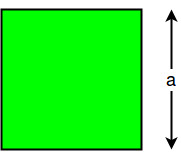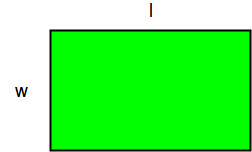# Program to find Perimeter / Circumference of Square and Rectangle

The circumference of a figure is the sum of all the side lengths. To calculate the circumference of square, length of one of the side is required as all sides are equal. To calculate the circumference of rectangle, length and breadth of rectangle is required.

Circumference of a Square:The circumference of a square is given by the formula:

```C = 4 * a
where a is the side length.
```

Examples :

```input: 4
output: 16

input: 3
output: 12
```

## C++

 `// CPP program to find ` `// Circumference of a square ` `#include ` `using` `namespace` `std; ` ` `  `int` `Circumference(``int` `a) ` `{ ` `    ``return` `4 * a; ` `} ` ` `  `// Driver Code ` `int` `main() ` `{ ` `    ``int` `a = 5; ` `    ``cout << ``"Circumference of"` `         ``<<``" a square is "` `         ``<< Circumference(a); ` `    ``return` `0; ` `} ` ` `  `// This code is contributed  ` `// by mohitw16 `

## Java

 `// Java program to find ` `// Circumference of a square ` ` `  `import` `java.io.*; ` `class` `GFG  ` `{ ` `    ``int` `Circumference(``int` `a) ` `    ``{ ` `        ``return` `4` `* a; ` `    ``} ` ` `  `    ``// Driver code ` `    ``public` `static` `void` `main(String args[]) ` `    ``{ ` `        ``GFG obj = ``new` `GFG(); ` `        ``int` `a = ``5``; ` `        ``System.out.println(``"Circumference of "` `+  ` `                                ``"a square is "` `+  ` `                          ``obj.Circumference(a)); ` `    ``} ` `} ` ` `  `// This code is contributed ` `// by Anshika Goyal. `

## Python3

 `# Python3 Program to find  ` `# Circumference of a square ` ` `  `def` `Circumference(a): ` `        ``return` `(``4` `*` `a) ` ` `  `# Driver code ` `a ``=` `5` `c ``=` `Circumference(a) ` `print``(``"Circumference of a "` `+` `       ``"square is % d"` `%` `(c)) `

## C#

 `// C# program to find Circumference ` `// of a square ` `using` `System; ` ` `  `class` `GFG  ` `{ ` ` `  `    ``static` `int` `Circumference(``int` `a) ` `    ``{ ` `        ``return` `4 * a; ` `    ``} ` ` `  `    ``// Driver Code ` `    ``public` `static` `void` `Main() ` `    ``{ ` `        ``int` `a = 5; ` ` `  `        ``Console.WriteLine(``"Circumference"` `+  ` `                       ``" of a square is "` `+  ` `                         ``Circumference(a)); ` `    ``} ` `} ` ` `  `// This code is contributed by vt_m. `

## PHP

 ` `

Output :

```Circumference Of a square is 20
```

Circumference of a rectangle:The circumference of a rectangle is given by the formula:

```C = 2 * (l + W)
where l is the length and W is the width.
```

Examples :

```input: 2 4
output: 12

input: 4 6
output: 20
```

## C++

 `// C++ Program to find  ` `// Circumference of a rectangle ` `#include ` `using` `namespace` `std; ` ` `  `int` `Circumference(``int` `l, ``int` `w) ` `{ ` `    ``return` `(2 * (l + w)); ` `} ` ` `  `// Driver code ` `int` `main() ` `{ ` `    ``int` `l = 8, w = 4; ` ` `  `    ``int` `c = Circumference(l, w); ` ` `  `    ``cout << ``"Circumference of a"` `         ``<< ``" rectangle is "`  `         ``<< c << endl; ` ` `  `    ``return` `0; ` `} ` ` `  `// This code is contributed by vt_m. `

## Python3

 `# Python Program to find  ` `# Circumference of a rectangle ` ` `  `def` `Circumference(l, w): ` `        ``return` `(``2` `*` `(l ``+` `w)) ` ` `  `# Driver code ` `l ``=` `8` `w ``=` `4` `c ``=` `Circumference(l, w) ` `print``(``"Circumference of a"` `+` `  ``" rectangle is % d"` `%` `(c)) `

## Java

 `// java Program to find  ` `// Circumference of a rectangle ` `import` `java.io.*; ` ` `  `class` `GFG  ` `{ ` ` `  `    ``static` `int` `Circumference(``int` `l,  ` `                             ``int` `w) ` `    ``{ ` `        ``return` `(``2` `* (l + w)); ` `    ``} ` ` `  `    ``// Driver code ` `    ``static` `public` `void` `main(String[] args) ` `    ``{ ` `        ``int` `l = ``8``, w = ``4``; ` ` `  `        ``int` `c = Circumference(l, w); ` ` `  `        ``System.out.println(``"Circumference of "` `+ ` `                         ``"a rectangle is "` `+ c); ` `    ``} ` `} ` ` `  `// This code is contributed by vt_m. `

## C#

 `// C# Program to find  ` `// circumference of a rectangle ` `using` `System; ` ` `  `class` `GFG  ` `{ ` ` `  `    ``static` `int` `Circumference(``int` `l,  ` `                             ``int` `w) ` `    ``{ ` `        ``return` `(2 * (l + w)); ` `    ``} ` ` `  `    ``// Driver code ` `    ``static` `public` `void` `Main() ` `    ``{ ` `        ``int` `l = 8, w = 4; ` ` `  `        ``int` `c = Circumference(l, w); ` ` `  `        ``Console.WriteLine(``"Circumference of "` `+  ` `                        ``"a rectangle is "` `+ c); ` `    ``} ` `} ` ` `  `// This code is contributed by vt_m. `

## PHP

 ` `

Output :

```Circumference of a rectangle is 24
```

GeeksforGeeks has prepared a complete interview preparation course with premium videos, theory, practice problems, TA support and many more features. Please refer Placement 100 for details

My Personal Notes arrow_drop_upCheck out this Author's contributed articles.

If you like GeeksforGeeks and would like to contribute, you can also write an article using contribute.geeksforgeeks.org or mail your article to contribute@geeksforgeeks.org. See your article appearing on the GeeksforGeeks main page and help other Geeks.

Please Improve this article if you find anything incorrect by clicking on the "Improve Article" button below.

Improved By : vt_m, jit_t